### Home > CAAC > Chapter 13 > Lesson 13.RE2-S > Problem2-135

2-135.
1. Decide whether each of the following pairs of expressions or equations is equivalent for all values of x (or a and b). If they are equivalent, show how you can be sure. If they are not, justify your reasoning completely. Homework Help ✎

1. (x + 3)2 and x2 + 9

2. (x + 4)2 and x2 + 8x + 16

3. (x + 1)(2x − 3) and 2x2x − 3

4. 3(x − 4)2 + 2 and 3x2 − 24x + 50

5. (x3)4 and x7

6. ab2 and a2b2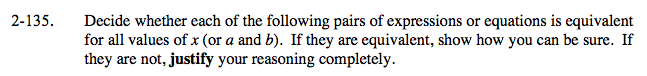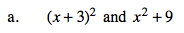Try substituting numbers for x and simplifying each expression. 0 and 1 can give different results, so check them as well as other numbers.

These two expressions are not equivalent. (But if you had only used 0 you might have thought they were).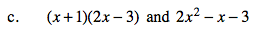Multiply and simplify.

These two expressions are equivalent.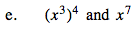See part (a).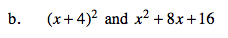Remember that (x + 4)2 means (x + 4)(x + 4) and multiply.

These two expressions are equivalent.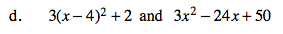Simplify the first expression and compare. Be sure you read the hint in part (b).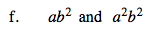Try substituting numbers for a and b.